## Convolutional Neural Network (CNN) basics

Welcome to part twelve of the Deep Learning with Neural Networks and TensorFlow tutorials. In this tutorial, we're going to cover the basics of the Convolutional Neural Network (CNN), or "ConvNet" if you want to really sound like you are in the "in" crowd.

The Convolutional Neural Network gained popularity through its use with image data, and is currently the state of the art for detecting what an image is, or what is contained in the image. CNNs even play an integral role in tasks like automatically generating captions for images.

The basic CNN structure is as follows: `Convolution -> Pooling -> Convolution -> Pooling -> Fully Connected Layer -> Output`

`Convolution` is the act of taking the original data, and creating feature maps from it.`Pooling` is down-sampling, most often in the form of "max-pooling," where we select a region, and then take the maximum value in that region, and that becomes the new value for the entire region. `Fully Connected Layers` are typical neural networks, where all nodes are "fully connected." The convolutional layers are not fully connected like a traditional neural network.

Okay, so now let's depict what's happening. We'll start with an image of a cat: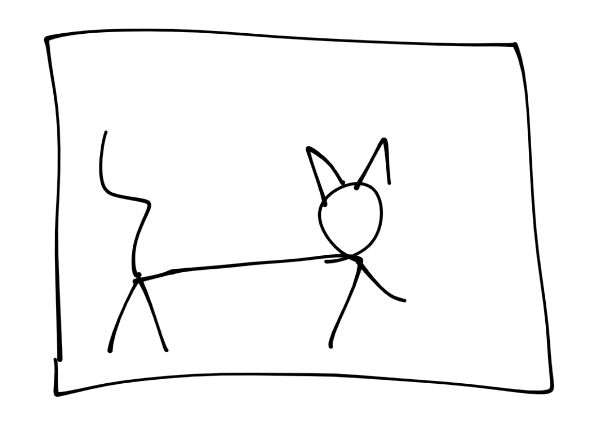Then "convert to pixels:"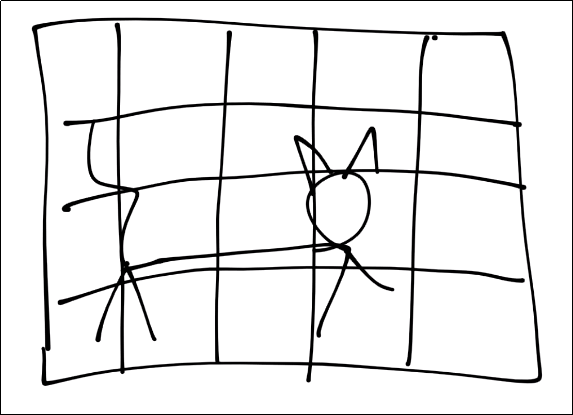For the purposes of this tutorial, assume each square is a pixel. Next, for the convolution step, we're going to take a certain window, and find features within that window: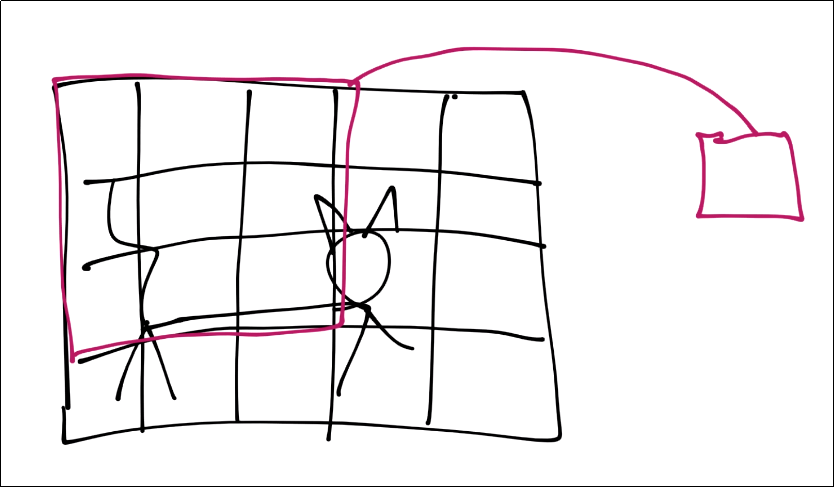That window's features are now just a single pixel-sized feature in a new featuremap, but we will have multiple layers of featuremaps in reality.

Next, we slide that window over and continue the process. There will be some overlap, you can determine how much you want, you just do not want to be skipping any pixels, of course.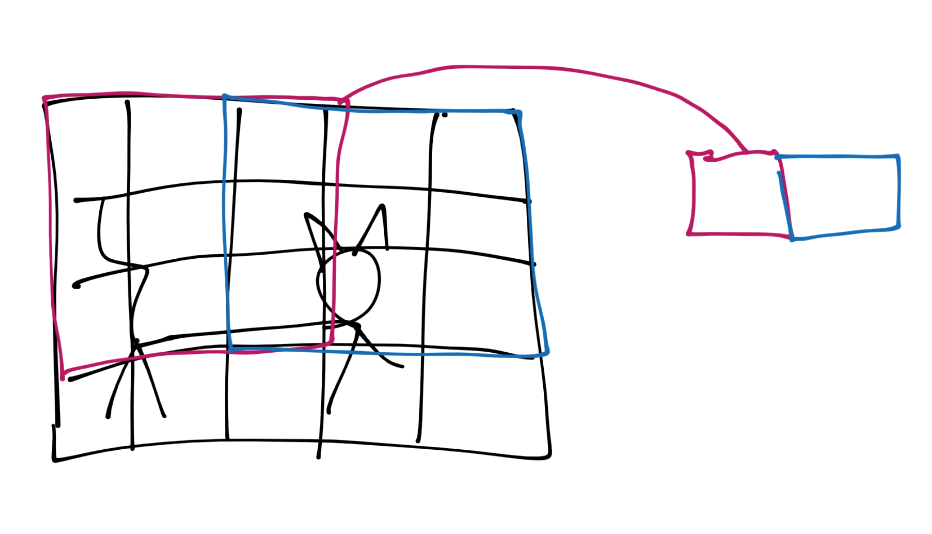Now you continue this process until you've covered the entire image, and then you will have a featuremap. Typically the featuremap is just more pixel values, just a very simplified one: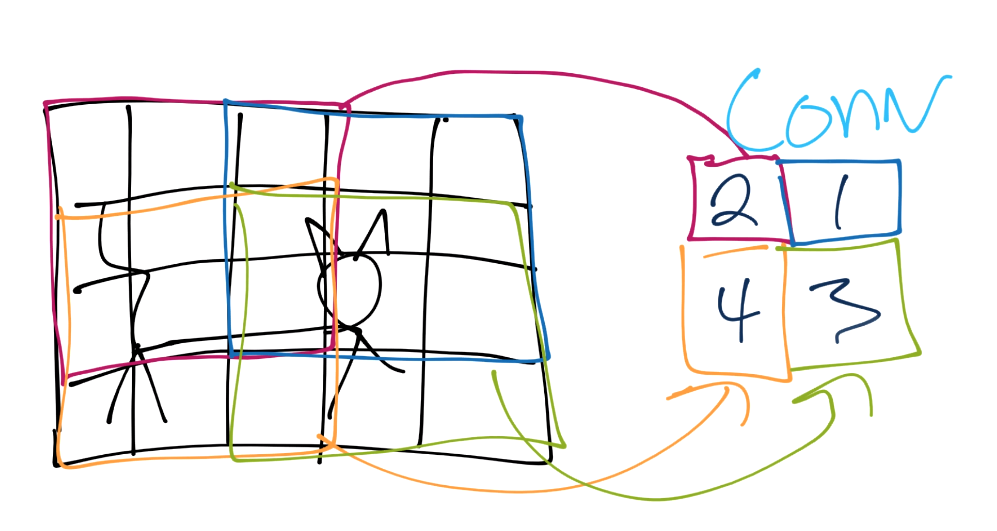From here, we do pooling. Let's say our convolution gave us (I forgot to put a number in the 2nd row's most right square, assume it's a 3 or less):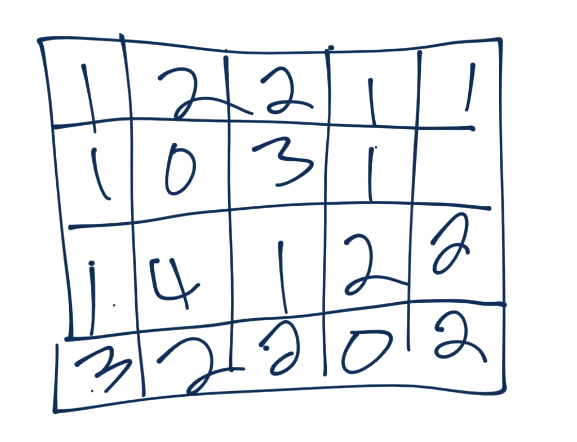Now we'll take a 3x3 pooling window: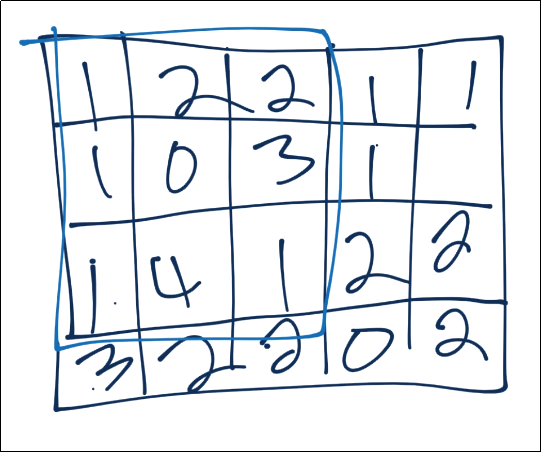The most common form of pooling is "max pooling," where we simple take the maximum value in the window, and that becomes the new value for that region.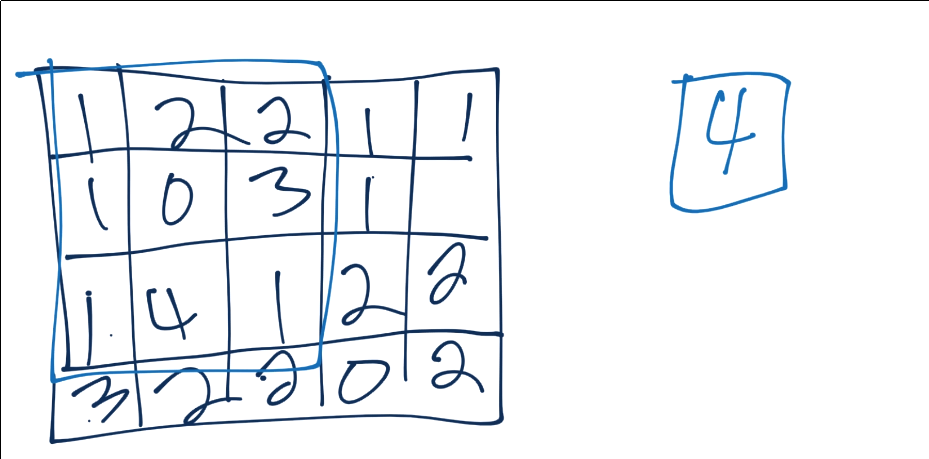We continue this process, until we've pooled, and have something like: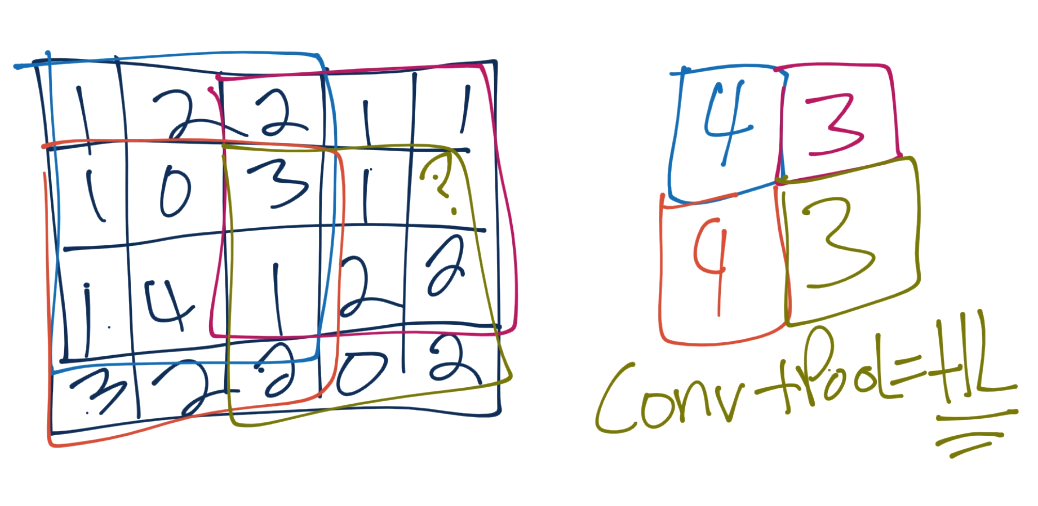Each convolution and pooling step is a hidden layer. After this, we have a fully connected layer, followed by the output layer. The fully connected layer is your typical neural network (multilayer perceptron) type of layer, and same with the output layer.

In the next tutorial, we're going to create a Convolutional Neural Network in TensorFlow and Python.

The next tutorial:• Practical Machine Learning Tutorial with Python Introduction

• Regression - Intro and Data

• Regression - Features and Labels

• Regression - Training and Testing

• Regression - Forecasting and Predicting

• Pickling and Scaling

• Regression - Theory and how it works

• Regression - How to program the Best Fit Slope

• Regression - How to program the Best Fit Line

• Regression - R Squared and Coefficient of Determination Theory

• Regression - How to Program R Squared

• Creating Sample Data for Testing

• Classification Intro with K Nearest Neighbors

• Applying K Nearest Neighbors to Data

• Euclidean Distance theory

• Creating a K Nearest Neighbors Classifer from scratch

• Creating a K Nearest Neighbors Classifer from scratch part 2

• Testing our K Nearest Neighbors classifier

• Final thoughts on K Nearest Neighbors

• Support Vector Machine introduction

• Vector Basics

• Support Vector Assertions

• Support Vector Machine Fundamentals

• Constraint Optimization with Support Vector Machine

• Beginning SVM from Scratch in Python

• Support Vector Machine Optimization in Python

• Support Vector Machine Optimization in Python part 2

• Visualization and Predicting with our Custom SVM

• Kernels Introduction

• Why Kernels

• Soft Margin Support Vector Machine

• Kernels, Soft Margin SVM, and Quadratic Programming with Python and CVXOPT

• Support Vector Machine Parameters

• Machine Learning - Clustering Introduction

• Handling Non-Numerical Data for Machine Learning

• K-Means with Titanic Dataset

• K-Means from Scratch in Python

• Finishing K-Means from Scratch in Python

• Hierarchical Clustering with Mean Shift Introduction

• Mean Shift applied to Titanic Dataset

• Mean Shift algorithm from scratch in Python

• Dynamically Weighted Bandwidth for Mean Shift

• Introduction to Neural Networks

• Installing TensorFlow for Deep Learning - OPTIONAL

• Introduction to Deep Learning with TensorFlow

• Deep Learning with TensorFlow - Creating the Neural Network Model

• Deep Learning with TensorFlow - How the Network will run

• Deep Learning with our own Data

• Simple Preprocessing Language Data for Deep Learning

• Training and Testing on our Data for Deep Learning

• 10K samples compared to 1.6 million samples with Deep Learning

• How to use CUDA and the GPU Version of Tensorflow for Deep Learning

• Recurrent Neural Network (RNN) basics and the Long Short Term Memory (LSTM) cell

• RNN w/ LSTM cell example in TensorFlow and Python

• Convolutional Neural Network (CNN) basics
• Convolutional Neural Network CNN with TensorFlow tutorial

• TFLearn - High Level Abstraction Layer for TensorFlow Tutorial

• Using a 3D Convolutional Neural Network on medical imaging data (CT Scans) for Kaggle

• Classifying Cats vs Dogs with a Convolutional Neural Network on Kaggle

• Using a neural network to solve OpenAI's CartPole balancing environment